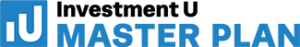# CAGR Calculator (Compound Annual Growth Rate)

The CAGR calculator below helps you figure out your average annual returns…

## Compound Annual Growth Rate Definition

The compound annual growth rate (CAGR) shows the annual rate of return of an investment over a certain period of time. It’s usually expressed in annual percentage terms.

The CAGR formula can be used to calculate the average growth of an investment. It can be helpful for investors to evaluate how their investments have performed over time. Comparing a stock’s CAGR to other stocks can help investors evaluate how well it performed.

Below is a CAGR calculator for anyone who wants to figure out their investment returns on a compound basis. Compound interest is a powerful concept to understand.

## CAGR Calculator Terms

The CAGR calculator requires three inputs: an investment’s beginning value, ending value and the number of years (or periods). As you enter the variables, the CAGR calculator automatically finds your compound annual growth rate results.

For a better understanding of the CAGR calculator inputs, here’s a breakdown…

Starting Value (\$) – the initial amount you put into your investment

Ending Value (\$) – the amount of your investment at the end

Number of Years (or Periods) – the numbers of years (or periods) the money is invested

Compound Annual Growth Rate Results – your average return percentage per year (or per period)

## CAGR Formula

This application bases its calculations on the compound annual growth rate formula (CAGR formula). If you know how to calculate the growth rate, you can determine the profit of your investment over a particular period. The CAGR formula is…

CAGR = ( EV / SV)^(1/N)-1

EV = Ending value (\$)

SV = Starting value (\$)

N = Number of years (or periods)

## CAGR Calculator Risks

Investment returns are volatile. They can vary significantly from one year to another. However, CAGR does not reflect volatility. One disadvantage of the CAGR calculator is that it assumes growth to be constant throughout the investment. A volatile investment may show real results that differ from this calculator.

For example, an investment could increase in value by 6% in a year. The next year, it could decrease in value by 3% and increase in value by 4% in the following year. The CAGR calculator will give a “smoothed” annual yield, so it can give the illusion that there is a steady growth rate. But investors should know the value of the underlying investment can be volatile and inconsistent.

Analyzing an investment’s annual return rate over time is important. To learn more about investing, subscribe to our free e-letter Investment U or these these other free e-letters. They’re packed with easy-to-understand insight from investing experts.

For the next step, you can check out our more detailed Investment Calculator. It’s also free, and it shows you how big your portfolio can grow after five, 10 or 20 years.##### Popular Posts

Become a smarter, more confident and more successful wealth builder with the free Investment U e-letter.